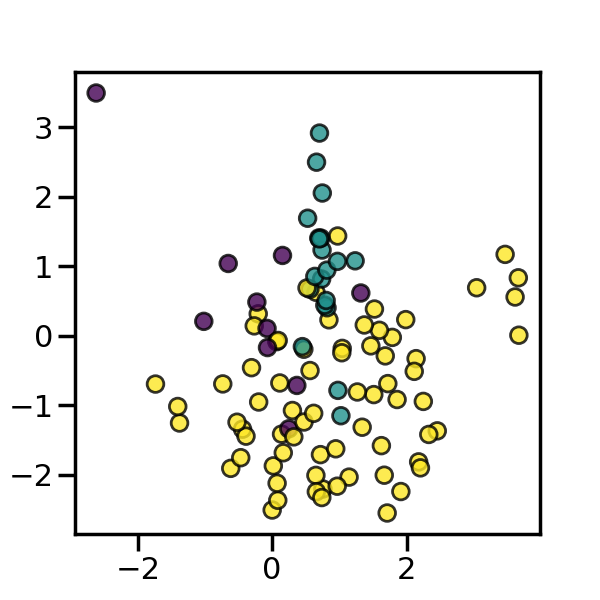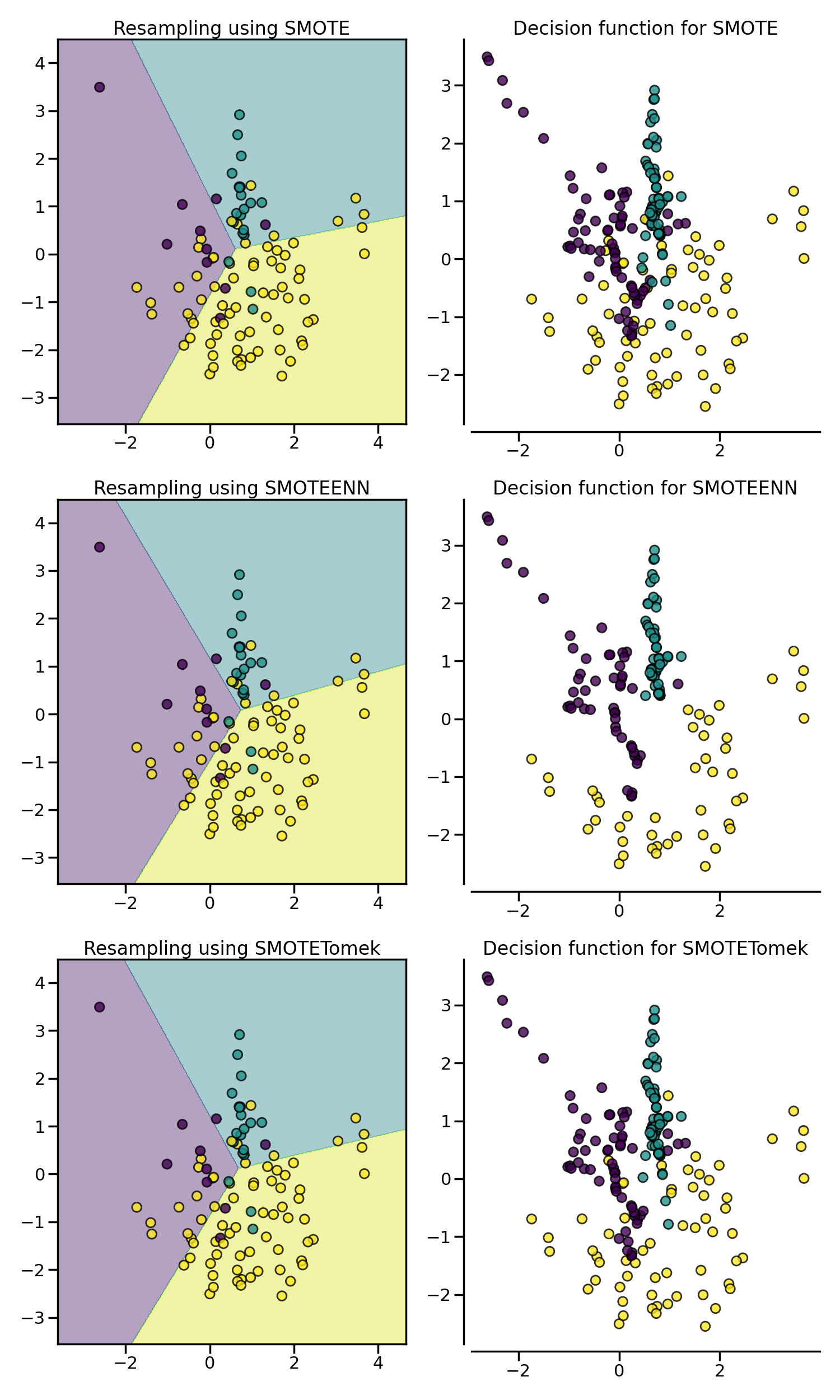# Compare sampler combining over- and under-sampling#

This example shows the effect of applying an under-sampling algorithms after SMOTE over-sampling. In the literature, Tomek’s link and edited nearest neighbours are the two methods which have been used and are available in imbalanced-learn.

```# Authors: Guillaume Lemaitre <g.lemaitre58@gmail.com>
```
```print(__doc__)

import matplotlib.pyplot as plt
import seaborn as sns

sns.set_context("poster")
```

## Dataset generation#

We will create an imbalanced dataset with a couple of samples. We will use `make_classification` to generate this dataset.

```from sklearn.datasets import make_classification

X, y = make_classification(
n_samples=100,
n_features=2,
n_informative=2,
n_redundant=0,
n_repeated=0,
n_classes=3,
n_clusters_per_class=1,
weights=[0.1, 0.2, 0.7],
class_sep=0.8,
random_state=0,
)
```
```_, ax = plt.subplots(figsize=(6, 6))
_ = ax.scatter(X[:, 0], X[:, 1], c=y, alpha=0.8, edgecolor="k")
```The following function will be used to plot the sample space after resampling to illustrate the characteristic of an algorithm.

```from collections import Counter

def plot_resampling(X, y, sampler, ax):
"""Plot the resampled dataset using the sampler."""
X_res, y_res = sampler.fit_resample(X, y)
ax.scatter(X_res[:, 0], X_res[:, 1], c=y_res, alpha=0.8, edgecolor="k")
sns.despine(ax=ax, offset=10)
ax.set_title(f"Decision function for {sampler.__class__.__name__}")
return Counter(y_res)
```

The following function will be used to plot the decision function of a classifier given some data.

```import numpy as np

def plot_decision_function(X, y, clf, ax):
"""Plot the decision function of the classifier and the original data"""
plot_step = 0.02
x_min, x_max = X[:, 0].min() - 1, X[:, 0].max() + 1
y_min, y_max = X[:, 1].min() - 1, X[:, 1].max() + 1
xx, yy = np.meshgrid(
np.arange(x_min, x_max, plot_step), np.arange(y_min, y_max, plot_step)
)

Z = clf.predict(np.c_[xx.ravel(), yy.ravel()])
Z = Z.reshape(xx.shape)
ax.contourf(xx, yy, Z, alpha=0.4)
ax.scatter(X[:, 0], X[:, 1], alpha=0.8, c=y, edgecolor="k")
ax.set_title(f"Resampling using {clf.__class__.__name__}")
```

`SMOTE` allows to generate samples. However, this method of over-sampling does not have any knowledge regarding the underlying distribution. Therefore, some noisy samples can be generated, e.g. when the different classes cannot be well separated. Hence, it can be beneficial to apply an under-sampling algorithm to clean the noisy samples. Two methods are usually used in the literature: (i) Tomek’s link and (ii) edited nearest neighbours cleaning methods. Imbalanced-learn provides two ready-to-use samplers `SMOTETomek` and `SMOTEENN`. In general, `SMOTEENN` cleans more noisy data than `SMOTETomek`.

```from sklearn.linear_model import LogisticRegression

from imblearn.combine import SMOTEENN, SMOTETomek
```
```from imblearn.over_sampling import SMOTE
from imblearn.pipeline import make_pipeline

samplers = [SMOTE(random_state=0), SMOTEENN(random_state=0), SMOTETomek(random_state=0)]

fig, axs = plt.subplots(3, 2, figsize=(15, 25))
for ax, sampler in zip(axs, samplers):
clf = make_pipeline(sampler, LogisticRegression()).fit(X, y)
plot_decision_function(X, y, clf, ax)
plot_resampling(X, y, sampler, ax)
fig.tight_layout()

plt.show()
```Total running time of the script: (0 minutes 2.539 seconds)

Estimated memory usage: 11 MB

Gallery generated by Sphinx-Gallery